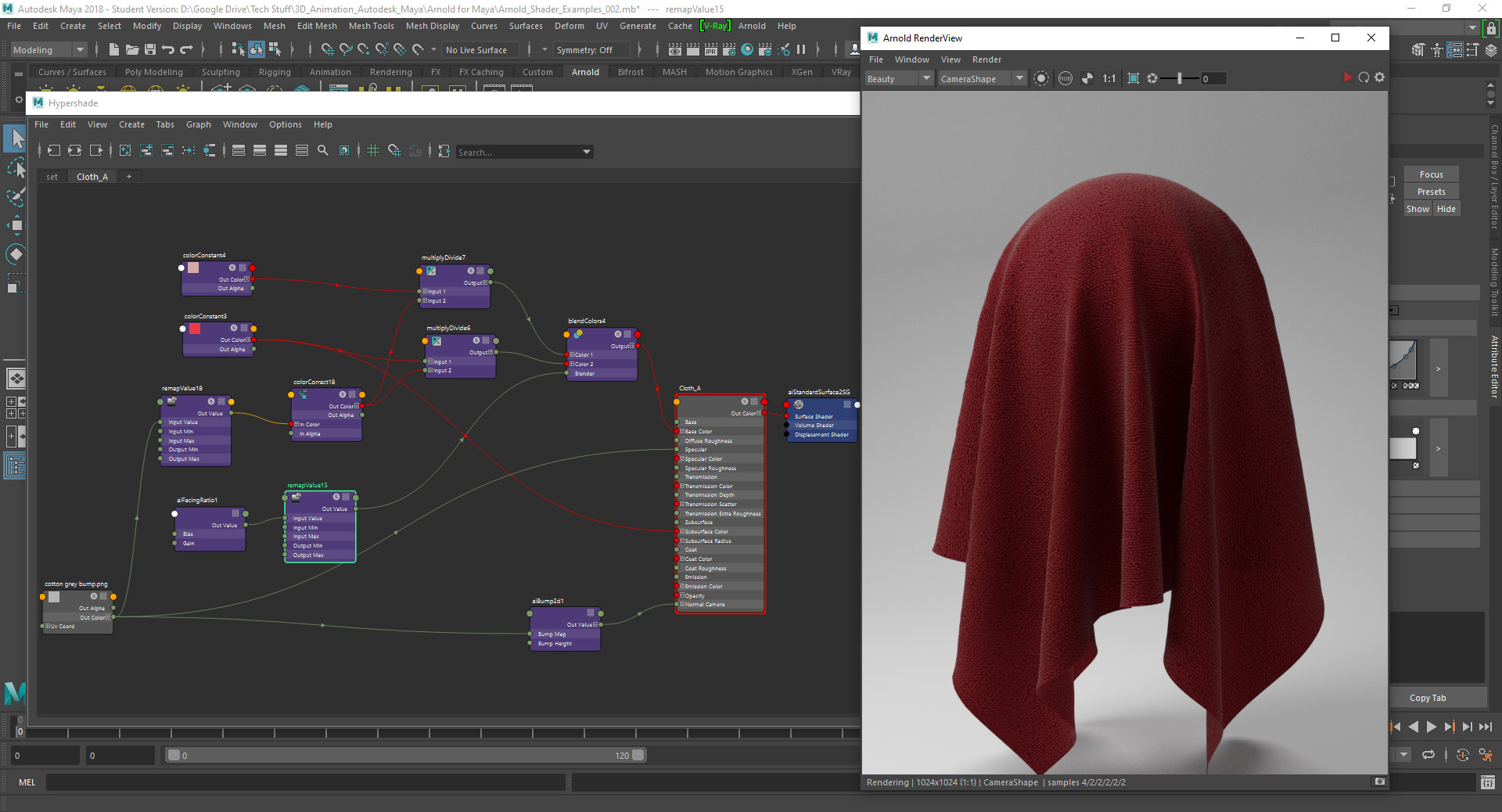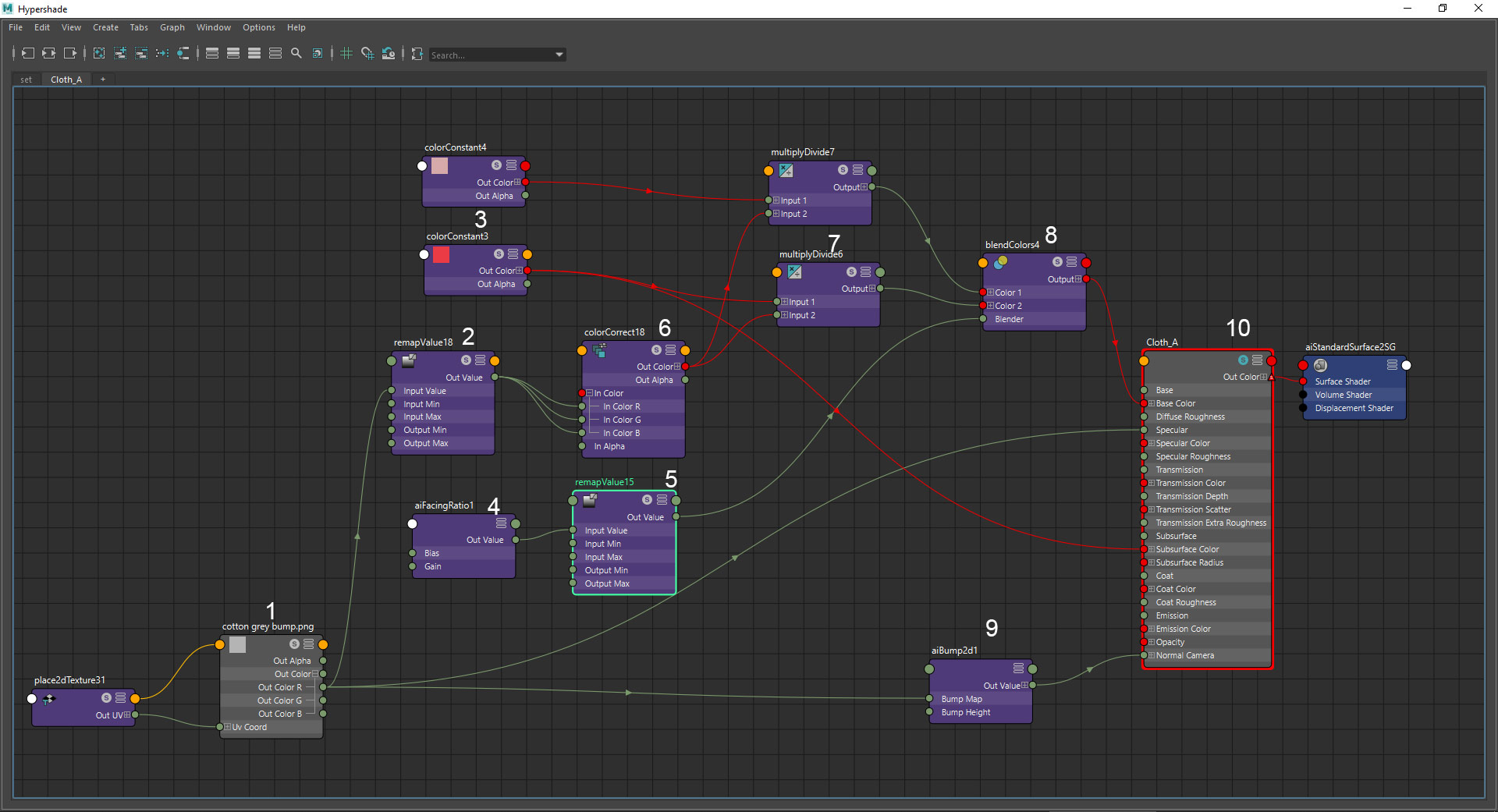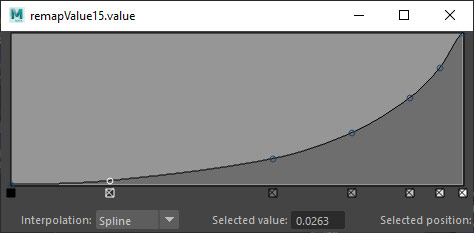## Writing a basic OSL color shader

The following is an introduction to basic OSL shader syntax using a simple color blending shader example. a more general explanation of the subject can be read here.

Notes:
> It’s highly recommended to get acquainted with basic C language syntax, since it’s the basis for common shading languages like OSL, HLSL and GLSL.
> More detailed information about writing OSL shaders can be found in the osl-languagespec PDF document from ImageWorks’s OSL GitHub.

This example shader blends 2 color sources according to the surface viewing angle (aka “facing ratio” or “incident angle” or “Perpendicular-Parallel”). the user can choose a facing (“front”) color or texture, a side color or texture, and the shader’s output bell be a mix of the these 2 inputs that depends on the angle the surface is viewed at.

``` #include "stdosl.h"

[[ string help = "Blend colors by view angle" ]]
(
color facing_color = color(0, 0, 0)
[[ string help = "The color (or texture) that will appear at perpendicular view angle" ]],
color side_color = color(1, 1, 1)
[[ string help = "The color (or texture) that will appear at grazing view angle" ]],
float base_blend = 0.0
[[ string help = "The percent of side_color that is mixed with facing_color at perpendicular view angle",
float min = 0.0, float max = 1.0]],
float curve_exponent = 1.0
[[ string help = "A power exponent value by which the blend value is raised to control the blend curve",
float min = 0.001, float max = 10.0]],
output color color_out = color(1, 1, 1))
{
// calculate the linear facing ratio:
float facing_ratio = acos(abs(dot(-I,N))) / M_PI_2;
// calculate the curve facing ratio:
float final_blend_ratio = pow(facing_ratio , curve_exponent);
// blend the facing color:
color final_facing_color = (facing_color * (1 - base_blend)) + (side_color * base_blend);
// blend and output the final color:
color_out = ((final_facing_color * (1 - final_blend_ratio)) + (side_color * final_blend_ratio));
}```

The first statment:

`#include "stdosl.h"`

The #include statement is a standard C compiler directive to link the OSL source code library code file stdosl.h to the shader’s code, so that the OSL data types and functions in the code will be recognized.
* Some systems compile the code successfully without this statement. I’m not sure if their compiler links stdosl.h automatically or not.

The double-square bracketed statements provide both help annotations and value range limits for the shader parameters:

`[[ string help = "The percent of side_color that is mixed with facing_color at perpendicular view angle", float min = 0.0, float max = 1.0]]`

Note that these statements are appended to single parameters in the shader right before the comma character that ends the parameter statement.
* Not all shading systems that supprt OSL also implement the annotations in the shader’s interface generated by the host software (the shader will work, but it’s parameters wont be described and limited to the defined value range).

Removing the #include statement, annotations and comments,
We can see that the OSL shader structure is very similar to a C function:

```shader cglColorAngleBlend
(
color facing_color = color(0, 0, 0),
color side_color = color(1, 1, 1),
float base_blend = 0.0,
float curve_exponent = 1.0,
output color color_out = color(1, 1, 1)
)
{
float facing_ratio = acos(abs(dot(-I,N))) / M_PI_2;
float final_blend_ratio = pow(facing_ratio , curve_exponent);
color final_facing_color = (facing_color * (1 - base_blend)) + (side_color * base_blend);
color_out = ((final_facing_color * (1 - final_blend_ratio)) + (side_color * final_blend_ratio));
}```

First the data type, in this case shader, followed by the shader identifier, in this case “cglColorAngleBlend”:

`shader cglColorAngleBlend`

After the shader’s type and identifier, a list of parameters is defined within parentheses, separated by comma’s. these parameters define the shader’s input’s, outputs, and default values. Output parameters are preceded by the output modifier.

(
<parameter type> <parameter identifier> = <parameter default value>,
<parameter type> <parameter identifier> = <parameter default value>,
output <parameter type> <parameter identifier> = <parameter default value>
)

```(
color facing_color = color(0, 0, 0),
color side_color = color(1, 1, 1),
float base_blend = 0.0,
float curve_exponent = 1.0,
output color color_out = color(1, 1, 1)
)```

In this case the shader has 4 user input parameters, and 1 output parameter.
2 color type parameters, “facing_color” and “side_color” for the facing and side color that will be blended together, a float* parameter “base_blend” that specifies how much of the side color will be mixed with the facing color regardless of view angle, and a second float parameter “curve_exponent” specifying a power exponent by which the blend value will be raised to create a non-linear blend curve.
The output parameter “color_out” is a color that will calculated by the shader.
* Note that even though the the output parameter will be calculated by the shader, it is required to define a default value for it for the shader to compile.

After the shader parameters, enclosed within curly braces is the actual body of the shader code, containing the instructions, each ending by a semicolon ; character:

{
<instruction>;
<instruction>;
…..
}

```{
float facing_ratio = acos(abs(dot(-I,N))) / M_PI_2;
float final_blend_ratio = pow(facing_ratio , curve_exponent);
color final_facing_color = (facing_color * (1 - base_blend)) + (side_color * base_blend);
color_out = ((final_facing_color * (1 - final_blend_ratio)) + (side_color * final_blend_ratio));
}```

I the case of our shader the first code instruction is to define a new float internal variable named “facing_ratio”, calculate the surface/view angle and assign the resulting value to it:

`float facing_ratio = acos(abs(dot(-I,N))) / M_PI_2;`

The expression:

acos( abs( dot( -I, N ) ) )  / M_PI_2

calculates incident angle, i.e. angle between 2 vectors, originating at the surface shading point, one pointing towards the origin of the incoming ray, and the other the surface normal, as a factor of 0 to 1 representing 0 – 90 degrees.
These 2 vector are easily obtained through the built in OSL global variables N and I. N is the surface normal at the shading point, and I is the incoming ray vector pointing to the shading point which is inverted in this case to point backwards by  typing a minus before it: -I.
The incident angle is calculated in radians as the arc-cosine of the dot-product of N and -I and then divided by half a π to convert it to a linear factor of 0 to 1 representing 0 to 90 degrees in radians, M_PI_2 being a convenient half π constant.
* M_PI being a full π, M_2PI being 2π representing 180 degrees in radians and 360 degrees respectively (OSL provides there are more constants in this series).

The second instruction raises the facing ratio that was calculated in the previous instruction by a power value provided by the curve_exponent input parameter, to create a non linear angle/color blend in values other than 1.0.
The resulting modified blend value is stored in a new internal variable final_blend_ratio:

`float final_blend_ratio = pow(facing_ratio , curve_exponent);`

Note:
We could avoid setting a new variable by modifying the value of  the facing_ratio variable, and we could also combine the these 2 instruction into 1 bigger expression like this:

pow( acos( abs( dot( -I, N ) ) ) / M_PI_2 , curve_exponent )

But I decided to keep it separated into 2 variable and 2 instructions for clarity.
* try modifying the code as an exercise

The third instruction modifies the input color facing_color by premixing it with the input side_color according to the percent give by the input parameter base_blend and assigns the resulting color to a new internal variable named final_facing_color:

`color final_facing_color = (facing_color * (1 - base_blend)) + (side_color * base_blend);`

The expression:

( facing_color * ( 1 – base_blend ) ) + ( side_color * base_blend )

Calculates a linear combination** (linear interpolation) between the 2 input colors using the base_blend as a 0 – 1 factor between them.
* Note that OSL allows to define arithmatic operations freely between colors and floats.

The forth and final instruction creates the final mix between the modified facing color stored in final_facing_color variable and the side color given by the input color parameter side_color, by again, calculating a linear combination between the 2 colors, this time using final_blend_ratio variable value we calculated previously as the combination factor, and very importantly, finally, assigning the mixed result to the shader output parameter color_out so it will be the final output of the shader:

`color_out = ((final_facing_color * (1 - final_blend_ratio)) + (side_color * final_blend_ratio));`

This screen capture shows this shader at work in Blender and Cycles, connected to a Principled BSDF shader as it’s base color source:Thats it! 🙂

Clarifications:

* A “float” data type is simply the the computer-science geeky way of saying “accurate non-integer number”. when we have to store numbers that can describe geometry and color, we need a data type that isn’t limited to integers so for that purpose we use float values. there’s actually a lot more to the float formal definition in computer science, but for our purpose here this will suffice.

** A Linear Combination, or Linear interpolation (lerp) is one of the most useful numerical operations in 3D geometry and color processing (vector math):
A * ( 1 – t ) +  B * t
A and B being your source and target locations or colors or any other value you need to interpolate and t being the blending factor from 0 – 1.

Related posts:

## Basic Cloth Material in Arnold for MayaSoftware:
Maya 2018 | Arnold 5

An example of a basic traditional (not scanned) cloth material setup in Arnold 5 for Maya using an aiStandardSurface shader.The shading network uses a classic angle dependent color blend to simulate the color of the cloth being washed out at grazing angle of view.

Explanation of the node graph:1. A black and white fabric weave texture that will serve as input for multiple shading channels.
* This is actually not the best example of such a pattern, and could be replaced with a much better texture.2. A remapValue node is used to set contrast to the fabric pattern (reduce contrast in this case) prior to it being multiplied with the fabric colors.
* Note that only one of the textures RGB channels is connected to the remapValue node since it’s a float (mono) processor and not RGB.* Note that depending on the fabric texture, you may have to design different curves to achieve the right effect.
3. Two colors are defined with colorConstant nodes:
A deep color as the main fabric color, and a washed out color for grazing angle view (“side color”).
4. An aiFacingRatio node is used as an input for incident angle info.
* Note that in this case I checked the node’s invert option to make it behave more like other systems I’m used to (if you don’t use invert, the angle blend curve in 5 will be different..)
5.  A remapValue node used to set the angle blend curve or in other words, how much does the color appears washed out per change of view angle of the cloth surface.
* The longer it take the curve to become steep from left to right, the more the main color will be dominant before the washed out color will appear.6. A colorCorrect node is used in this example just as a way to convert the remapped float value back to RGB for being multiplied with the cloth colors.
* We could also connect it directly to the individual float components of the RGB colors but this way the node graph is cleaner.
7. A multiplyDivide node is used to multiply the processed fabric texture with the 2 fabric colors “baking” the pattern into the color.
8. A blendColors node is used to blend the 2 processed fabric colors together according to the processed facingRatio angle input.
The result is the final cloth color that is connected to the aiStandardSurface shader.
9. An aiBump2d node is used to convert the fabric pattern to normal data that will be connected to the aiStandardSurface shader to produce bumps.
10. An aiStandartSurface shader serving as the main shading node for this material.
* Note that under Geometry the Thin Walled option is checked so that the Subsurface layer of the shader will act as a Paper Shader rather than SSS.
* The main cloth color is connected to the SubSurface Color input.